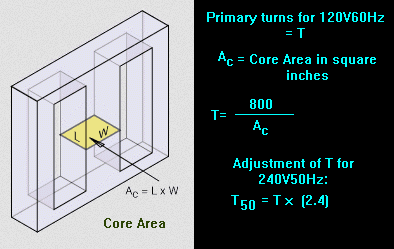The minimum number of turns required for a 120V primary of a 60Hz power transformer is a closely guarded secret.Not really, but everyone always talks about turns ratios, but forgets to mention the minimum number of turns for the primary. Well, the iron/silicon/metal core of the transformer can accept only so much magnetic flux before it saturates and can't take more. If you go beyond this, the inductance drops a lot and you end up drawing a lot more current off the powerline and it will get really hot, not good. A conservative rule of thumb, for transformer laminations you may salvage from a junked 60Hz transformer: Number of turns needed for the 120V60Hz primary = 800/(area of the core in square inches). You measure the height (in the below diagram "L") of the pile of laminations, and the width ("W") of the center leg of the E lamination. In other words, imagine a single turn of wire tight on the center core, the area of the loop this single turn forms is the area. In the below diagram, it's L times W. Do not include the outer legs of the E, or the I. A bigger area will make for a lower number of turns. Once you have the number of primary turns, then you can do the turns ratio to get the number of turns for the secondary. Add a few more turns to make up for resistance of the wire voltage losses. If your powerline frequency is 50Hz, you need 60/50 times the above 120V60Hz result for your primary for 120V, and twice that for 240V. Which works out to be 2.4 times the above 120V60Hz result aboveOf course, you also need to select magnet wire thick enough to handle the current the load will impose, but thin enough so you can get all the turns around the core without getting too fat that the outside parts of the E lams won't fit over. And also allow for insulation to insulate the core lams from the bottom of the windings, and insulation between the primary and the secondary (safety regs want 1400VDC of insulation) and the sides of the windings too. Winding the turns neatly will allow tighter packing of the turns). If the wire works out to be too thin for the currents, you will need a bigger core. This equation should get you in the ballpark:A is the core cross section in sq inches and W is the VoltAmp output.

A more general equation:E is the winding voltage, ie, primary input voltage, or a secondary output voltage.
F is the powerline (mains) frequency
H is the number of lines of magnetic flux per square inch of iron, for most power transformers it's 56300 RMS. The iron won't accept much more.
N is the number of turns for the above mentioned E primary or secondary.
A is the cross section area in sq inches of the core

If E is taken as 1 it gives the turns per volt for any winding on the core.

Working this equation to check if it matches the simple rule of thumb:
Do some algebra, set A to 1 square inch, F to 60Hz, E to 120V, and H to 56300, and we have

N=(108 E)/(A H F (4.44)) = (108 *120)/(1 * 56300 * 60 * (4.44)) = 800. But this is circular fudging, as I got the 56300 working the equation the other way.

High permeability iron alloys used in transformers reach magnetic saturation at 1.6 to 2.2 teslas (T). Okay, a telsa is the number of lines of magnetic flux per square meter. There's 1553 square inches in a square meter. Taking the above 56300 * 1552 = 87377600. But the winding voltage E is an RMS value, and the magnetic saturation mentioned would be a limit, and you have to take into account of the peak value of E. Recalculating the H fudge variable with the peak value of E instead of its RMS value gets us 78400 per square inch for H. Which gets us 123000000 for square meters. Combining it with the 108 in the denominator in the above equation gets us 1.23, which looks like it may be telsas. If so, this looks like a conservitive value to stay well away from saturation. All this handwaving may or may not be valid, as I'm no physicist.But if my handwaving is good, it looks to validate the rule of thumb "Number of turns needed for the 120V60Hz primary = 800/(area of the core in square inches)".